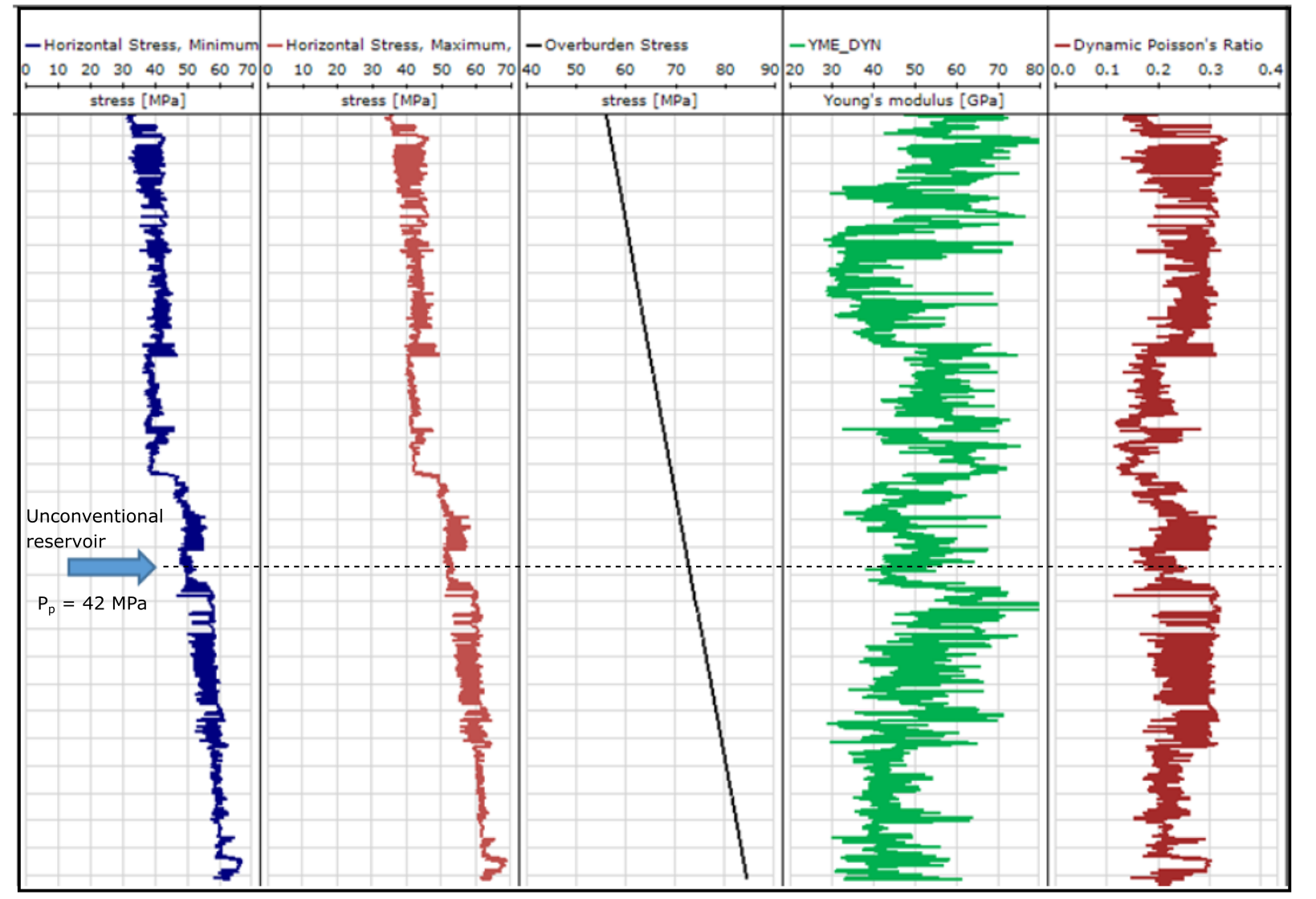# 2.4 Problems

1. Compute the vertical stress gradient resulting from a carbonate rock made of 70% dolomite and 30% calcite, porosity 10% and filled with brine 1,060 kg/m. Look up for mineral densities in the web in trusted sources. Provide answer in psi/ft, MPa/km, and ppg.

2. Calculate (without the help of a computer) the total vertical stress in an off-shore location at 10,000 ft of total depth (from surface) for which: water depth is 1,000 m (with mass density 1,030 kg/m), bulk mass density of rock at the seabed is 1,800 kg/mincreasing linearly until a depth of 500 m below sea-floor to 2,350 kg/mand relatively constant below 500 m below seafloor. Why would rock bulk mass density increase with depth?

3. The following table contains the estimated bulk mass densities for an offshore location in Brazil as a function of true vertical depth sub sea (TVDSS). Water depth is 500 m. Measurements indicate that porosity of shale layers estimated through electrical resistivity measurements.
1. Plot the profiles ofv.s. depth (MPa v.s. m and ft v.s psi)
2. Plot the profile of (hypothetical) hydrostatic water pressure. Assume the density of brine is 1,031 kg/min the rock pore space (MPa v.s. m and ft v.s. psi).
3. Additional compaction lab measurements on shale cores indicate a good fitting of the porosity-effective vertical stress relation through Equation 2.19, with parametersandMPa. Estimate the actual pore pressure in the shale. Is there overpressure? At what depth does it start?
4. According to porosity measurements, does vertical effective stress always increase with depth? Justify.

Note: You are encouraged to summarize all calculations in a single plot as a function of depth (inverted vertical axis): hypothetical pore pressure, actual pore pressure and total vertical stress.

 Depth Bulk mass density Shale porositymkg/mWater 0 1025 NA Water 100 1026 NA Water 200 1026 NA Water 300 1030 NA Water 400 1030 NA Water 500 1031 NA Sand 600 1900 NA Sand 700 2190 NA Sand 800 2200 NA Sand 900 2230 NA Sand 1000 2235 NA Sand 1100 2240 NA Shale 1200 2275 0.305 Shale 1300 2305 0.297 Shale 1400 2310 0.286 Shale 1500 2308 0.281 Shale 1600 2310 0.285 Shale 1700 2305 0.293 Shale 1800 2310 0.307 Shale 1900 2324 0.305 Shale 2000 2319 0.298

4. Go to https://github.com/dnicolasespinoza/GeomechanicsJupyter and download the files “HCLonghorn.las” and “HCdeviationsurvey.dev”. The files include the well logging data and the well trajectory of a well for the Longhorn Field near Plaquemines Parish, Louisiana. The oilfield is an onshore oilfield. The first one is a well logging file (.las). You will find here measured depth (DEPTH [ft] - Track 1) and bulk mass density (ZDNC [g/cc] - Track 27). The second file has the deviation survey of the well. Column 3 is measured depth (MD), column 4 is TVDSS, column 5 is the E-W offset from the surface location, and column 6 is the N-S offset from the surface location. The water depth at this well location is 38 ft. You may assume an average bulk mass density of 2 g/cc between the surface and the beginning of the bulk density data.
1. (optional) Plot the 3-D well trajectory and wellbore direction in a lower hemisphere projection (plot inclination angles and azimuth angles in a polar coordinate system) using the deviation survey.
2. Plot TVDSS [ft] as a function of MD [ft]. For this simple trajectory you may use a linear fit to relate TVDSS and MD.
3. Plot bulk density (x-axis) verse TVDSS (ft) (y-axis).
4. Compute and plot the total vertical stress (psi) (x-axis) versus TVDSS (ft) (y-axis). Compute and plot also the expected hydrostatic pore pressure.

5. Based on the US stress map published by the USGS (Fig. 2.24 - https://www.usgs.gov/media/images/new-us-stress-map), answer the following for the Anadarko Basin near mid-center Oklahoma:
1. What is the predominant direction of?.
2. What is the predominant stress regime?
3. What would the ideal orientation of a hydraulic fracture be in this area?

6. The following image shows a “stress log” for a location in the Permian Basin (onshore):1. What is the approximate range of depth shown in the stress log?
2. What is the approximate depth at the location of the unconventional reservoir (answer specifically at the location of the dashed line - same for following questions)?
3. What are the minimum and maximum total horizontal stresses?
4. Is the reservoir pressure “hydrostatic”? What is the effective vertical stress?
5. What is the stress regime?

Note: You may use a plot digitizer (for example, https://apps.automeris.io/wpd/) to obtain numerical data from image files.